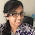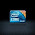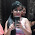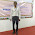# GATE Questions-DBMS-Functional Dependency

Previous GATE questions with solutions on DBMS (Functional Dependency) - CS/IT

GATE-1999
1. Let R= (A, B, C, D, E, F) be a relation scheme with the following dependencies: C->F, E->A, EC->D, A->B. Which of the following is a key for R?
(a) CD             (b) EC           (c) AE            (d) AC

Ans: option (b)
Explanation:
Find the closure set of all the options given. If any closure covers all the attributes of the relation R then that is the key.
Algorithm to find Closure Set
Step1: Equate an attribute or attributes to X for which closure needs to be identified.
Step2: Take each FD (functional dependency) one by one and check whether the left side of FD is available in X, if yes then add the right side attributes to X if it is not available.
Step3: Repeat step 2 as many times as possible to cover all FD's.
Step4: After no more attributes can be added to X declare it as the closure set.

FDs: C->F, E->A, EC->D, A->B
Find closure set for CD.
X = CD
= CDF {C->F}
No more attributes can be added to X. Hence closure set of CD = CDF
Find closure set for EC.
X = EC
= ECF {C->F}
= ECFA {E->A}
Closure set of EC covers all the attributes of the relation R.

GATE-2000
2. Given the following relation instance.
-------
X  Y  Z
-------
1  4  2
1  5  3
1  6  3
3  2  2
-------
Which of the following functional dependencies are satisfied by the instance?
(a) XY -> Z and Z -> Y
(b) YZ -> X and Y -> Z
(c) YZ -> X and X -> Z
(d) XZ -> Y and Y -> X

Ans: option (b)
Explanation:
Association among attributes is known as Functional Dependencies (FD). A FD X->Y require that the value of X uniquely determines the value of Y where X and Y are set of attributes.
For example,
Roll_No -> Name: the value of Roll_No uniquely determines the Name.
Roll_No, Book_No -> Issue_Date : In the case of library, Roll_No and Book_No can determine the Issue_Date of a book.

In option (a), its given Z->Y, it means that the value of Z uniquely determines the value of Y. But here the value 2 of Z, gives two different values of Y i.e. 4 and 2. Therefore this FD is not satisfied by the instance.
In option (c), its given X->Z, it means that the value of X uniquely determines the value of Z. But here the value 1 of X, gives two different values of Z i.e. 2 and 3. Therefore this FD is not satisfied by the instance.
n option (d), its given Y->X, here the value of Y uniquely determines the value of X. Therefore this FD is satisfied by the instance. Now take FD XZ->Y, here (1,3) cannot uniquely determine the value of Y. (1,3) gives two values for Y i.e. 5 and 6. Therefore this FD (XZ->Y) is not satisfied by the instance.

GATE-2002
3. From the following instance of a relational schema R(A, B, C), we can conclude that:
----------
A   B   C
----------
1   1   1
1   1   0
2   3   2
2   3   2
----------
(a) A functionally determines B and B functionally determines C
(b) A functionally determines B and B does not functionally determine C
(c) B does not functionally determine C
(d) A does not functionally determine B and B does not functionally determine C

Ans: option (c)
Explanation:
Looking into an instance we can't conclude that A functionally determines B. A->B could hold true to the given instance but not on the entire database. But we can be sure that B does not functionally determine C because for the value 1 of  B, it gives two different values of C i.e. 1 & 0.
Issue in option (d) - Again from the provided instance we cannot say that A does not determine B. A->B may or may not hold. Therefore only possible option is (c).

GATE-2005
4. Consider a relation scheme R = (A, B, C, D, E, H) on which the following functional dependencies hold: {A–>B, BC–>D, E–>C, D–>A}. What are the candidate keys of R?
(a) AE, BE
(b) AE, BE, DE
(c) AEH, BEH, BCH
(d) AEH, BEH, DEH

Ans: option (d)
Explanation:
If you focus on the right side of the functional dependencies you can see that E & H cannot be derived using the left side of functional dependencies. Hence E & H will definitely be part of the candidate key.  Therefore only option (d) satisfies such condition.
If any closure includes all attributes of a table then it becomes the candidate key. Find closure (As explained in question 1) of AEH as below.
Closure of AEH = AEHB   {A->B}
= AEHBC  {E->C}
= AEHBCD {BC->D}

GATE-2005(IT)
5. In a schema with attributes A, B, C, D and E, following set of functional dependencies are given:
A->B
A->C
CD->E
B->D
E->A
Which of the following functional dependencies is NOT implied by the above set?
(a) CD->AC            (b) BD->CD         (c) BC->CD          (d) AC->BC

Ans: option (b)
Explanation:
For every options given, find the closure set of left side of each FD. If the closure set of left side contains the right side of the FD, then the particular FD is implied by the given set.
Option (a): Closure set of CD = CDEAB. Therefore CD->AC can be derived from the given set of FDs.
Option (c): Closure set of BC = BCDEA. Therefore BC->CD can be derived from the given set of FDs.
Option (d): Closure set of AC = ACBDE. Therefore AC->BC can be derived from the given set of FDs.
Option (b): Closure set of BD = BD. Therefore BD->CD cannot be derived from the given set of FDs.

GATE-2006
6. The following functional dependencies are given:
AB->CD, AF->D, DE->F, C->G , F->E, G->A
Which one of the following options is false?
(a)CF+ = {ACDEFG}                             (b)BG+ = {ABCDG}
(c)AF+ = {ACDEFG}                             (d)AB+ = {ABCDFG}

Ans: option(c)
Explanation:
AF+ = {AFDE}
As explained in question 1, find the closure set of each options.
Option (d) is also false. AB+ = {ABCDG}.

GATE-2013
Linked question ( 7 & 8 )

Relation R has eight attributes ABCDEFGH. Fields of R contain only atomic values.
F={CH->G, A->BC, B->CFH, E->A, F->EG} is a set of functional dependencies (FDs) so that F + is exactly the set of FDs that hold for R.

7. How many candidate keys does the relation R have?
(a) 3    (b) 4    (c) 5    (d) 6

Ans: option (b)

Explanation:

In a relational database, a key helps to uniquely identify each record within a table . A key is a combination of one or more fields/attributes in a table. If a relational schema has multiple keys,each key is a candidate key. One of the candidate keys is chosen  as the primary key.

To find the candidate keys, we need to find the closure of each attribute. (If x ia an attribute(field), set of attributes determined by x under a set F of functional dependencies is the closure of x under F, denoted x+ ).

Thus,

A+:ABCFHGE

B+: BCFHEGA

C+:C

D+:D

E+: EABCFHG
F+:FEGABCH
G+:G
H+ : H
A+,B+,E+,F+ contains all attributes except D. Thus there are 4 candidate keys DA,DB,DE and DF.

8. The relation R is
(a) in 1NF, but not in 2NF.
(b) in 2NF, but not in 3NF.
(c) in 3NF, but not in BCNF.
(d) in BCNF.

Ans: option (a)
Explanation:
An attribute that does not occur in any candidate key is called a non-prime attribute.
(Reference :https://en.wikipedia.org/wiki/Candidate_key)

Consider F->G; G is a non-prime attribute and F is a proper subset of a candidate key (refer the above question). This is a case of partial dependency. Hence 2NF condition is violated. similarly  A->C  and B->CFH also violates 2NF condition, hence R is not in 2NF.

Since attributes of relation R has only atomic values, R is in 1NF.

GATE - 2015
9. Consider the relation X(P, Q, R, S, T, U) with the following set of functional dependencies
F = {
{P, R} → {S, T}
{P, S, U} → {Q, R}
}
Which of the following is the trivial functional dependency in F+ is closure of F?
(a) {P, R} → {S, T}
(b) {P, R} → {R, T}
(c) {P, S} → {S}
(d) {P, S, U} → {Q}

Ans: option (c)
Explanation:
A functional dependency X → Y is trivial, if Y is a subset of X.
In the above question , {S}is a subset of {P,S}. Hence option (c) is the answer.

GATE - 2014
10. Consider the relation scheme R=(E,F,G,H,I,J,K,L,M,N) and the set of functional dependencies
{{E,F}→{G},{F}→{I,J},{E,H}→{K,L},{K}→{M},{L}→{N}}
on R. What is the key for R?
(a) {E,F}
(b) {E,F,H}
(c) {E,F,H,K,L}
(d) {E}

Ans: option (b)
Explanation:
Find closure set of all options. But as you can notice that H cannot be derived from any of the above functional dependencies it means that H should be present in the key. Therefore we need to check only the closure set of option b and option c which contains H.
Since EFH+ derives all the attributes in the relation R it is the candidate key. Note that option (c) is the super key since adding zero or more attributes to candidate key generates super key.

1.Hi,
For Q.No :3 - How option b got eliminated ?
For A It gives value 1 and 3 for the values 1 and 2 respectively . So It also determines B uniquely .so A functionally determines B also should be right .Please Correct me if am wrong .

1.It was a typing mistake Malini. As u said correct option is (b). Thank you for your note. Correction has been made.

2.hi,
For the 6th question I think AB closure will have only {ABCDG }.Please correct me if am wrong

1.Yes, option (d) is also false. AB closure = {ABCDG}. Thanks for this note... :)

2.so , AF+ = adef ??

3.in ques how C option is wrong?

1.Can you plz provide the question number..

4.Good work ....

5.6.thanku

7.8.for question 3 c) shud be answer, we cant tell what is determining what until we know entire schema, we can only tell negative,, this is a negative test

9.Excellent explanations

10.Fantastically fabulous....keep it uppppppp...

11.Superb explanation...simple and step by step...nice work...

12.13.faboulous explaination step by step ..very nice

14.for question number 3 correct answer is "option C".

15.Que3. I think correct option is C.
Looking into an instance of Relation we can't say A determines B.
We can be sure of what is not functionally determined by other.But we can't say about what
is determined by other. We don't know complete DB.and By the way FDs are made prior to
Relations.

16.Could anyone more explain Ques no. 8. I didn't get why option (a) is right.

1.Consider F->G; G is a non-prime attribute and F is a proper subset of a candidate key. This is a case of partial dependency. Hence 2NF condition is violated.
Similarly A->C and B->CFH also violates 2NF condition, hence R is not in 2NF.
Since it is given that attributes of relation R has only atomic values, R is in 1NF.

17.relation r=( a b c d e g) having functional dependencies F=(a->b, bg->e,c->d,d->g) find the candidate key
what is the answer of this question and give explanation for same

1.I think answer should be "ac" because clouser of (ac)+ = {abcdeg}
Explaination:
ac -> ac
ac -> acb(by a)
ac -> abcd(by c)
ac -> abcdg(by d)
ac -> abcdge(by bg)

18.